# Outline¶

• Bayesian learning

• repeated actions, observe each other
• ==> DeGroot model

• repeated communication, "naive" updating

# Outline: DeGroot Model¶

• ==> Basic Definitions

• When is there convergence?

• When is there a consensus?

• Who has influence?

• When is the consensus accurate

# Network structure and Learning¶

• Repeated communication

• Information comes only once

• See how information disseminates

• Who has influence, convergence speed, network structure impact...

# Bounded Rationality Model¶

• Repeatedly average beliefs of self with neighbors

• Non-Bayesian if weights do not adjust over time

• Can under-weight neightbors (just as in experiments)

# DeGroot (1974) Social Interaction Model¶

• Individuals {1,...n}

• T trust matrix, weighted directed network, stochastic matrix, $$\sum_j T_{ij} = 1, T_{ij} \ge 0$$

• somebody that never listens to anybody would have: $$T_{ii} = 1$$
• Start with beliefs, attitude, etc. $b_i(0)$ in [0,1]

• can also have these be vectors...
• Belief at time t updating: $$b_i(t) = \sum_j T_{ij} b_j(t-1)$$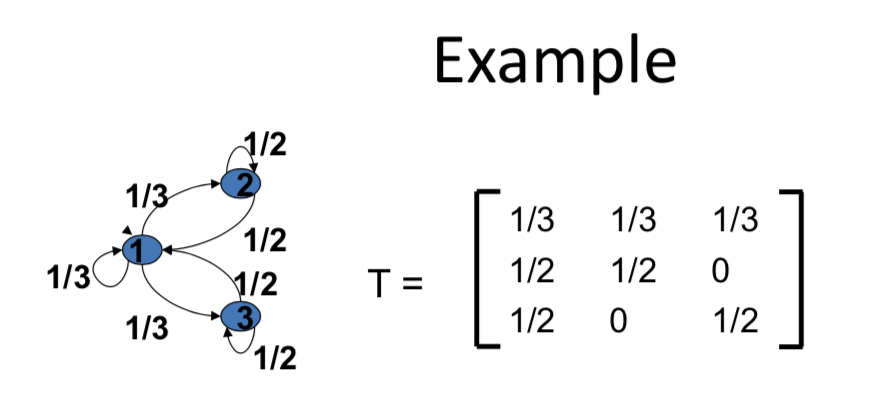So suppose that the initial beliefs of the three individuals were person "1" started with a belief of "0", person 3 started with a belief of "0", and it was only person "2" that started with a positive belief

• $b_1(0) = 0$, $b_2(0) = 1$, $b_3(0) = 0$
• $b_1(1) = 1/3$, $b_2(1) = 1/2$, $b_3(1) = 0$
• $b_i(1) = \sum_j T_{ij}*b_j(0)$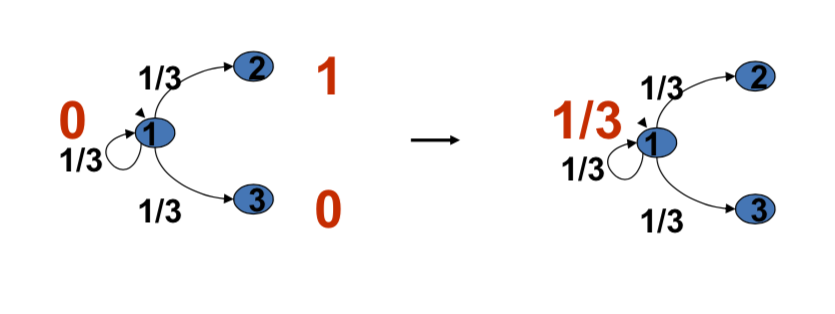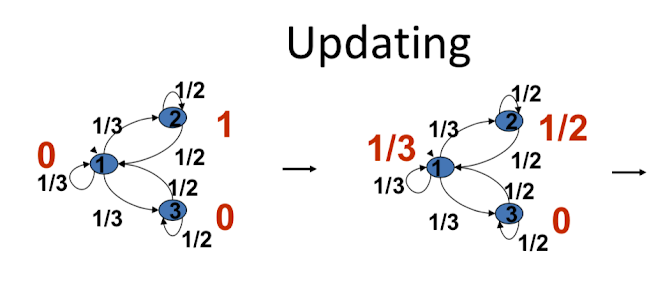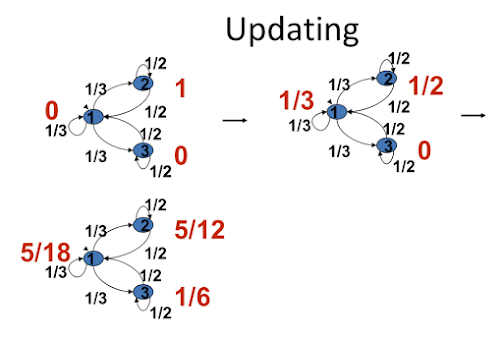eventually three individuals converge to "2/7"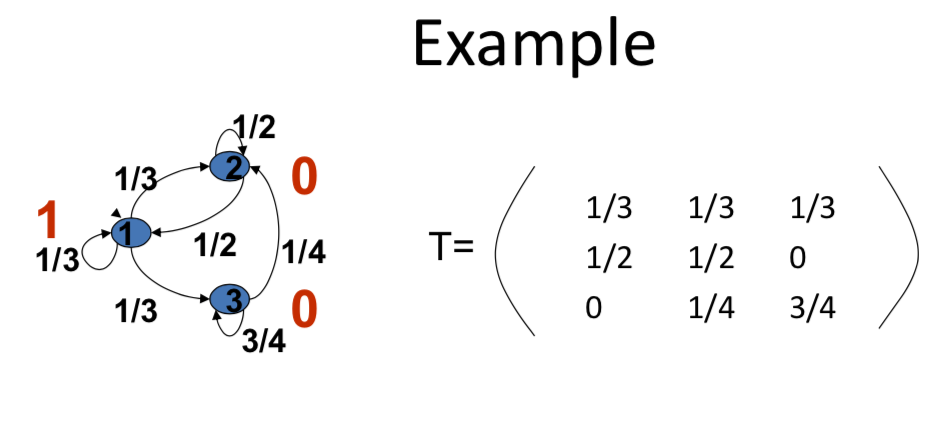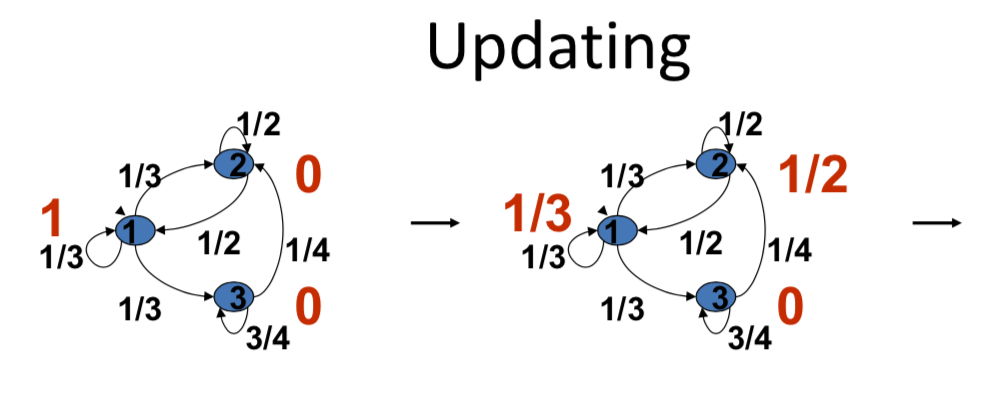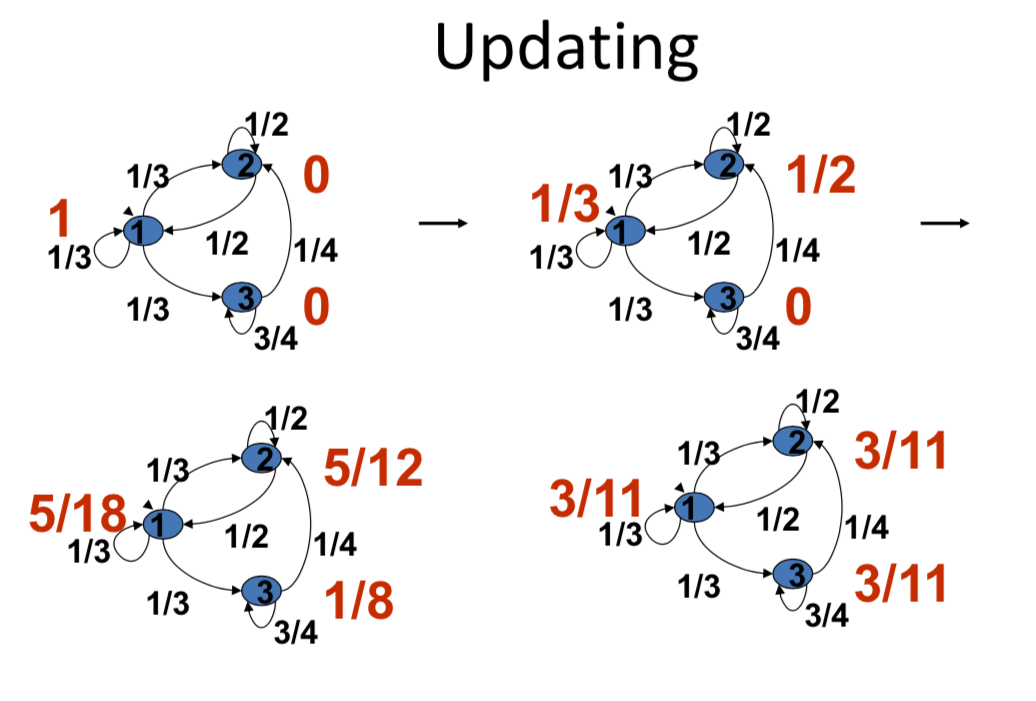It's essentially weighted averaging over time, so it's a nice linear system. And this linear system is something that we know a lot about, in terms of the mathematics. And these things are going to converge and we'll have a nice way of talking about what do these numbers turn out to be, how do they depend on the initial beliefs and how do they depend on the network. So there'll be an explicit solution that we can just calculate out directly in terms of what those numbers are and what those relations [INAUDIBLE] So that's going to be a nice part of the model, is we'll, we'll learn where, what's the limit point of this process. And we can actually also say a lot about the speed of convergence here and how that depends on the network and so forth.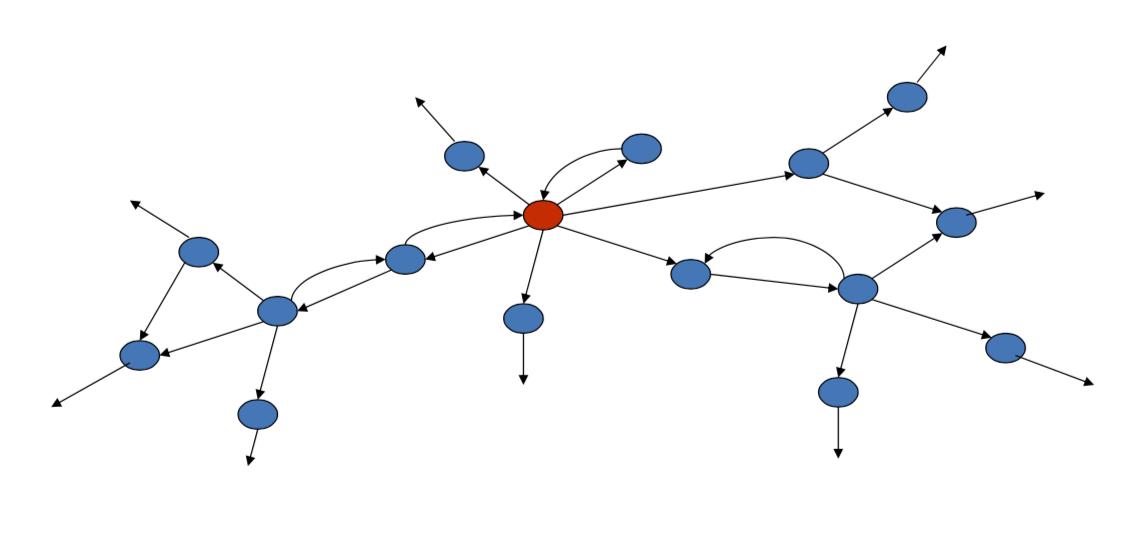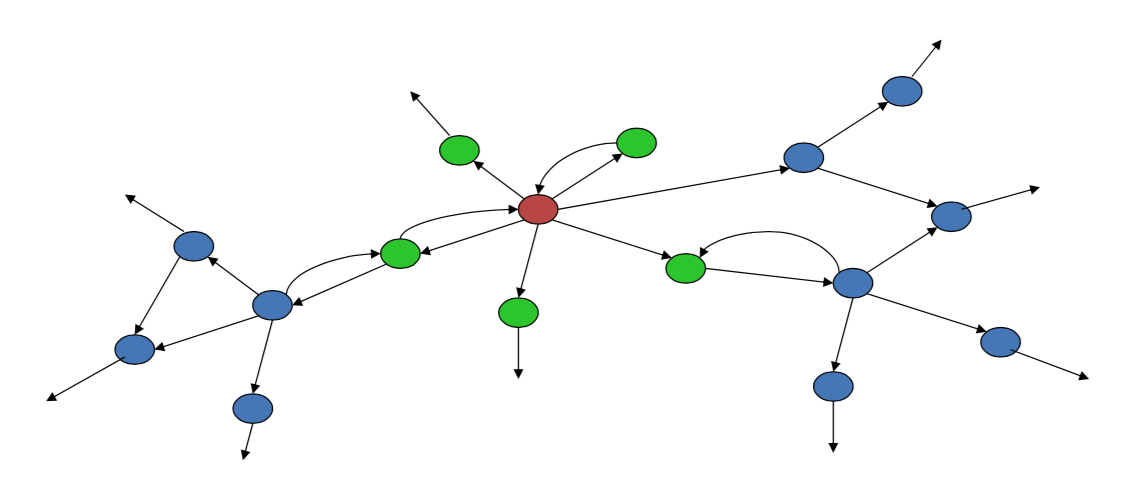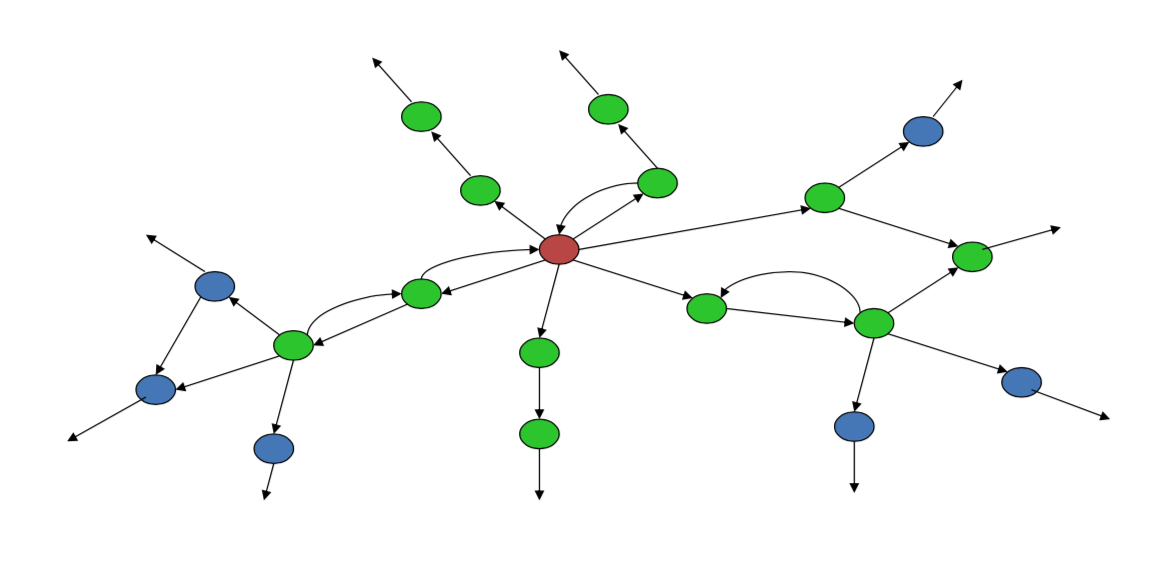so what's happening here is, you know, when a person talks to their friends effectively, you know, after one period they've incorporated information from these people. After 2 periods, they've i, indirectly incorporated information from 2 distances because this information passed on to people that were at distance 1 after, you know, the first period. And, and so, what happens is, is we end up getting if e, if these people are listening. To other individuals that information goes on and, and gets incorporated by the individual over time. So, as time goes on we've got more reach in terms of where the information is coming in from and the fact that people are averaging things means that we're going to end up with a nice conversion here so, things it's going to have nice properties.

# Other interpretations¶

• Social influence on actions

• Random actions (Markov process...)

• Page ranks...